International
Tables for
Crystallography
Volume D
Physical properties of crystals
Edited by A. Authier

International Tables for Crystallography (2006). Vol. D, ch. 2.2, pp. 303-304

## Section 2.2.12. The linearized augmented plane wave method

K. Schwarza*

aInstitut für Materialchemie, Technische Universität Wien, Getreidemarkt 9/165-TC, A-1060 Vienna, Austria
Correspondence e-mail: kschwarz@theochem.tuwein.ac.at

### 2.2.12. The linearized augmented plane wave method

| top | pdf |

The electronic structure of solids can be calculated with a variety of methods as described above (Section 2.2.11). One representative example is the (full-potential) linearized augmented plane wave (LAPW) method. The LAPW method is one among the most accurate schemes for solving the effective one-particle (the so-called Kohn–Sham) equations (2.2.10.3)and is based on DFT (Section 2.2.10) for the treatment of exchange and correlation.

The LAPW formalism is described in many references, starting with the pioneering work by Andersen (1975) and by Koelling & Arbman (1975), which led to the development and the description of the computer code WIEN (Blaha et al., 1990; Schwarz & Blaha, 1996). An excellent book by Singh (1994) is highly recommended to the interested reader. Here only the basic ideas are summarized, while details are left to the articles and references therein.

In the LAPW method, the unit cell is partitioned into (non-overlapping) atomic spheres centred around the atomic sites (type I) and an interstitial region (II) as shown schematically in Fig. 2.2.12.1. For the construction of basis functions (and only for this purpose), the muffin-tin approximation (MTA) is used. In the MTA, the potential is assumed to be spherically symmetric within the atomic spheres but constant outside; in the former atomic-like functions and in the latter plane waves are used in order to adapt the basis set optimally to the problem. Specifically, the following basis sets are used in the two types of regions:

• (1) Inside the atomic sphere t of radius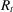(region I), a linear combination of radial functions times spherical harmonics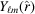is used (we omit the index t when it is clear from the context):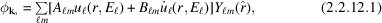whererepresents the angles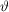andof the polar coordinates. The radial functions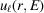depend on the energy E. Within a certain energy range this energy dependance can be accounted for by using a linear combination of the solution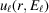and its energy derivative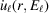, both taken at the same energy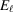(which is normally chosen at the centre of the band with the corresponding-like character). This is the linearization in the LAPW method. These two functions are obtained on a radial mesh inside the atomic sphere by numerical integration of the radial Schrödinger equation using the spherical part of the potential inside sphere t and choosing the solution that is regular at the origin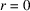. The coefficients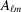and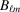are chosen by matching conditions (see below).Figure 2.2.12.1 | top | pdf |Schematic partitioning of the unit cell into atomic spheres (I) and an interstitial region (II).
• (2) In the interstitial region (II), a plane-wave expansion (see the Sommerfeld model, Section 2.2.5) is used: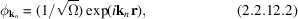where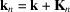,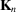are vectors of the reciprocal lattice,is the wavevector in the first Brillouin zone andis the unit-cell volume [see (2.2.5.3)]. This corresponds to writing the periodic function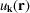(2.2.4.19)as a Fourier series and combining it with the Bloch function (2.2.4.18). Each plane wave (corresponding to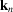) is augmented by an atomic-like function in every atomic sphere, where the coefficientsandin (2.2.12.1)are chosen to match (in value and slope) the atomic solution with the corresponding plane-wave basis function of the interstitial region.

The solutions to the Kohn–Sham equations are expanded in this combined basis set of LAPWs,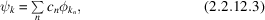where the coefficientsare determined by the Rayleigh–Ritz variational principle. The convergence of this basis set is controlled by the number of PWs, i.e. by the magnitude of the largestvector in equation (2.2.12.3).

In order to improve upon the linearization (i.e. to increase the flexibility of the basis) and to make possible a consistent treatment of semi-core and valence states in one energy window (to ensure orthogonality), additional (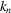-independent) basis functions can be added. They are called local orbitals' (Singh, 1994) and consist of a linear combination of two radial functions at two different energies (e.g. at the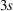and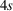energy) and one energy derivative (at one of these energies):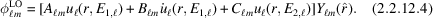The coefficients,and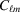are determined by the requirements that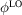should be normalized and has zero value and slope at the sphere boundary.

In its general form, the LAPW method expands the potential in the following form: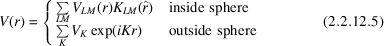where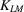are the crystal harmonics compatible with the point-group symmetry of the corresponding atom represented in a local coordinate system (see Section 2.2.13). An analogous expression holds for the charge density. Thus no shape approximations are made, a procedure frequently called the full-potential LAPW' (FLAPW) method.

The muffin-tin approximation (MTA) used in early band calculations corresponds to retaining only the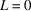and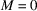component in the first expression of (2.2.12.5)and only the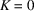component in the second. This (much older) procedure corresponds to taking the spherical average inside the spheres and the volume average in the interstitial region. The MTA was frequently used in the 1970s and works reasonable well in highly coordinated (metallic) systems such as face-centred-cubic (f.c.c.) metals. For covalently bonded solids, open or layered structures, however, the MTA is a poor approximation and leads to serious discrepancies with experiment. In all these cases a full-potential treatment is essential.

The choice of sphere radii is not very critical in full-potential calculations, in contrast to the MTA, where this choice may affect the results significantly. Furthermore, different radii would be found when one uses one of the two plausible criteria, namely based on the potential (maximum between two adjacent atoms) or the charge density (minimum between two adjacent atoms). Therefore in the MTA one must make a compromise, whereas in full-potential calculations this problem practically disappears.

### References

Andersen, O. K. (1975). Linear methods in band theory. Phys. Rev. B, 12, 3060–3083.
Blaha, P., Schwarz, K., Sorantin, P. I. & Trickey, S. B. (1990). Full-potential linearized augmented plane wave programs for crystalline systems. Comput. Phys. Commun. 59, 399–415.
Koelling, D. D. & Arbman, G. O. (1975). Use of energy derivative of the radial solution in an augmented plane wave method: application to copper. J. Phys. F Metal Phys. 5, 2041–2054.
Schwarz, K. & Blaha, P. (1996). Description of an LAPW DF program (WIEN95). In Lecture notes in chemistry, Vol. 67, Quantum-mechanical ab initio calculation of properties of crystalline materials, edited by C. Pisani. Berlin, Heidelberg, New York: Springer-Verlag.
Singh, D. J. (1994). Plane waves, pseudopotentials and the LAPW method. Boston, Dordrecht, London: Kluwer Academic Publishers.